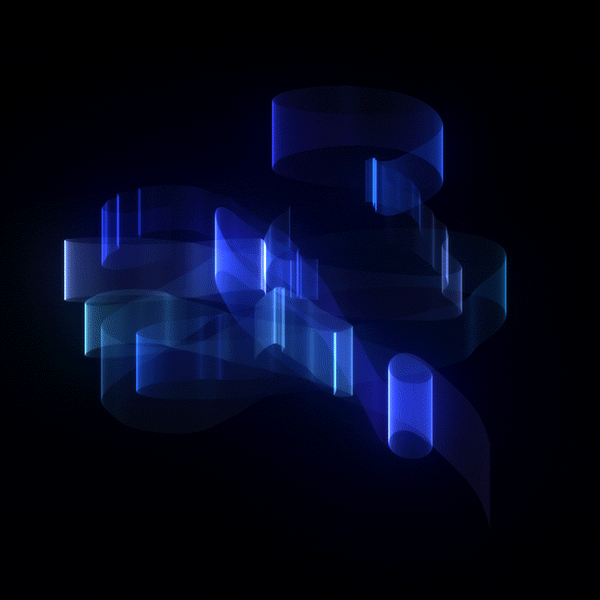A Probably Terrible Way to Render GradientsCrystal Schuller twitter logo github logo May 18・4 min read

My most recent project at 42 Silicon Valley has me creating a 3-D wireframe rendering engine in C. One of the required capabilities is to smoothly change the color of rendered lines according to their elevation, like this:So I needed to build a C function that can increment colors in a smooth gradient. It needed to work with Olivier Crouzet's miniLibX, which interprets RGB colors as a single hexadecimal int (which is probably what every graphics library does? I did not research this).

So we have one of these bois:

0xFFFFFF

who needs to turn into this boi:

0x2FA8F9

And it needs to look all pretty, like this:Having not done math since 2002, I had my work cut out for me.

I was floored to discover that these random jargon digits have actually been RGB number values the whole time, with each two-digit pair representing the value of red, green or blue light. That means hex codes can be broken down like this:Armed with this revelation, it was clear I needed to somehow isolate digit pairs in a hexadecimal number, manipulate them, and re-combine them.

Oh no, I realized, I have to do math with base-16. God, please, no...

No you don't, answered Beyoncé from on high via sunbeam, This will actually be super easy because bitshifting exists.

In decimal notation, 255 looks like this:
`255`

and in hexadecimal notation, it looks like this:
`FF`

but in binary, it looks like this:
`0000 0000 0000 0000 0000 0000 1111 1111`

Using the magic of bitwise operators, we can scooch this value over like this:
`FF << 8`

which results in this:
`0000 0000 0000 0000 1111 1111 0000 0000`

which in hexadecimal looks like this:
`FF00`

and that means we can do the same in reverse, which allows us to isolate the first two digits of a hexadecimal number like this:
`0x2FA8F9 >> 16 = 0x2F`
We have our R value!

Isolating the G and B values requires using a bitmask with the bitwise and, which Neso Academy can explain better than I can, but which looks like this:
`0x2FA8F9 & 0xFF = 0xF9`

In brief, it works like this:

0x2FA8F9 in binary is:
`0000 0000 0010 1111 1010 1000 1111 1001`
and 0xFF in binary is:
`0000 0000 0000 0000 0000 0000 1111 1111`

The bitwise and returns an integer containing the bits that both numbers have in common:
`0000 0000 0000 0000 0000 0000 1111 1001`

We can use a combination of these techniques to isolate the G value, so what we're left with is this:

`R = color >> 16`
`G = color >> 8 & 0xFF`
`B = color & 0xFF`

Now that we have all the color values isolated into separate variables, the math can begin.

The Math Begins

To create a gradient, we need to increment each color value by even increments, like this:The increment value is nice and easy to calculate:

`increment = (end_color - start_color) / steps`

`Steps` here are just pixels. Since my line-drawing algorithm is Not Fancy™, the number of pixels is just the larger of either x-distance or y-distance. We need the absolute (unsigned) values of these -- an x-change of -16 will evaluate as less than a y-change of 5, but 16 is the greater distance. I made a macro that finds the absolute max like this:

`steps = ABS_MAX((x1-x0), (y1-y0))`

Now that we have that, we can describe the color of each pixel as a function of three values: the `starting color`, the `increment`, and the `position` in the line.

`color = (position * increment) + start_color`

If we store `position` as a counter in our loop, we can find that piece easily, too.

```int position = 0; while(condition){ gradient_stuff(position); position++; }```

Now that we have all the pieces, we can create our gradient!

If we moved from our example colors 0xFFFFFF and 0x2FA8F9 over 5 steps, our increments would be:Here's what it looks like when we apply our color formula to the individual color channels at each step.You'll notice all these values are rounded to the nearest whole number, since RGB values can't handle decimals.

Once we have the individual values for R, G, and B, we can do the bitwise operations in reverse and add the values together to get the final color.

`RGB = (R << 16) + (G << 8) + B`
`RGB = (0x2F << 16) + (0xA8 << 8) + (F9)`
`RGB = 0x2F0000 + 0x00A800 + 0x0000F9`
`RGB = 0x2FA8F9`

Here's what that looks like:All of this has been done before, and probably a lot more elegantly and efficiently. But this function is my function and I made it all by myself, so I get a popsicle*. If you want to see more of this for some reason, you can view the rest of the project (still in progress) on github.
Here's the function in it's entirety.

``````# define R(a) (a) >> 16
# define G(a) ((a) >> 8) & 0xFF
# define B(a) (a) & 0xFF
# define RGB(a, b, c) ((a) << 16) + ((b) << 8) + (c)

int gradient(int startcolor, int endcolor, int len, int pix)
{
double increment;
int new;
int newcolor;

increment = (double)((R(endcolor)) - (R(startcolor))) / (double)len;
increment = (double)((G(endcolor)) - (G(startcolor))) / (double)len;
increment = (double)((B(endcolor)) - (B(startcolor))) / (double)len;

new = (R(startcolor)) + ft_round(pix * increment);
new = (G(startcolor)) + ft_round(pix * increment);
new = (B(startcolor)) + ft_round(pix * increment);

newcolor = RGB(new, new, new);

return (newcolor);
}
``````

* they do not actually give us popsicles.

DISCUSS (1)dev.to now has dark mode.

Select night theme in the "misc" section of your settings ❤️

Classic DEV Post from Jan 8

Smart Time Management and Productivity Guide via Weekly Reviews.